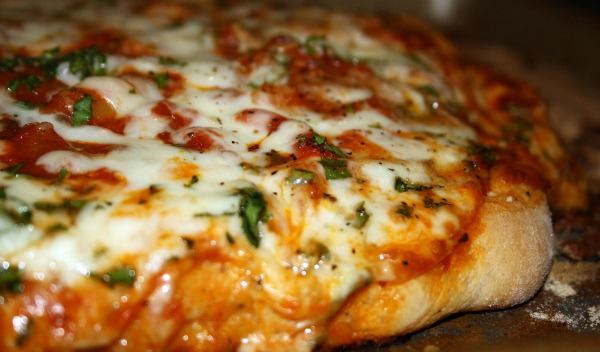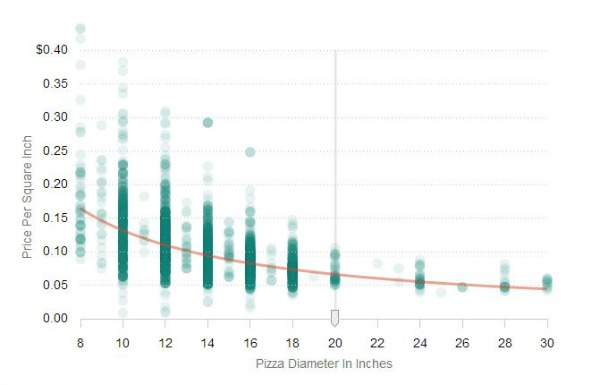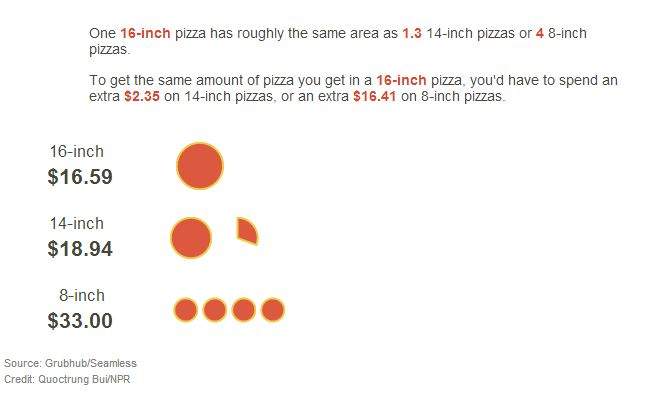Categories

Categories

## Mathematical Reasoning Explains Why You Should Always Order a Larger PizzaIn case you needed to be convinced to buy larger, cheesier pizzas, here’s some mathematical proof.

Using data on over 74,000 pizzas from 3,678 different US pizza joints, Quoctrung Bui of NPR created a graph illustrating the cost of pies versus the surface area of pies. What he discovered was something we’ve been sure of all along: the bigger the pizza, the cheaper the cost.The logic behind this is based on simple high school math: a pizza is a circle, a circle’s area increases with the square of the radius. For example, a 12-inch pizza is twice as large as an 8-inch pie, not just 4-inches more. On the other hand, an even better deal would be to go for the 16-inch, which is more than double the size of an 8-inch pie — about four times as large, in fact.

As you can see below, pizza prices don’t increase proportionally to the size of the pie.If you’re a bit dizzy from all the numbers, NPR’s interactive graph helps you visualize the concept.

In conclusion: Buy the large, then save any leftovers for the waffle iron.

Picthx NPR, British Mum